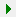Follow

# How do I create a multi-plot graph and export it to a JPG file in Grapher Scripter?

This article contains Grapher sample script that shows how to create a graph with line plots and bar charts and export to a JPG file.

To run this script:

2. Click Automation | Scripts | Run, select the BAS file from your downloads directory, and click Open.

OR:

1. Copy the script below.
2. Open Grapher and turn on the Script Manager by clicking View | Display | Script Manager.
3. Press Ctrl+A to select all of the existing lines in the Script Manager and then press DELETE.
4. Press Ctrl+V to paste it into the Script Manager.
5. Click the Start/Resume icon () in the Script Manager.

*********

```Sub Main
'Declares GrapherApp as an object
Dim GrapherApp As Object

'Creates an instance of the Grapher Application object
'and assigns it to the variable named "GrapherApp"
Set GrapherApp = CreateObject("Grapher.Application")

'Makes Grapher visible
GrapherApp.Visible = True

'Declares Plot as an object
Dim Plot As Object

'Creates a plot document in Grapher and
'assigns it to the variable named "Plot"
Set Plot = GrapherApp.Documents.Add(grfPlotDoc)

'Declares Graph as an object
Dim Graph As Object

'Creates a graph and assigns it to the
'variable named "Graph"
Set Graph = Plot.Shapes.AddLinePlotGraph(GrapherApp.Path+"\samples\tutorial.dat")

'Declare LinePlot as an object
Dim LinePlot As Object

'Set LinePlot to the first line plot on the "Graph" graph
Set LinePlot = Graph.Plots.Item(1)

'Add line plot from columns A/C

'Shorten Y Axis 1
Graph.Axes.Item("Y Axis 1").length = 3

'Add new Y Axis
Set YAxis2 = Graph.AddAxis(grfYAxis)

'Shorten Y Axis 2
YAxis2.length = 3

'Set axis position
YAxis2.PositionAxis(grfPositionRightTop, "Y Axis 1")

'Add Bar Chart using Y Axis 2
Graph.AddBarChart(GrapherApp.Path+"\samples\tutorial.dat",1,4, ,"Y Axis 2")

'Export to JPG
Plot.Export(GrapherApp.Path+"\samples\tutorial.jpg")
End Sub```

Updated October 8, 2018